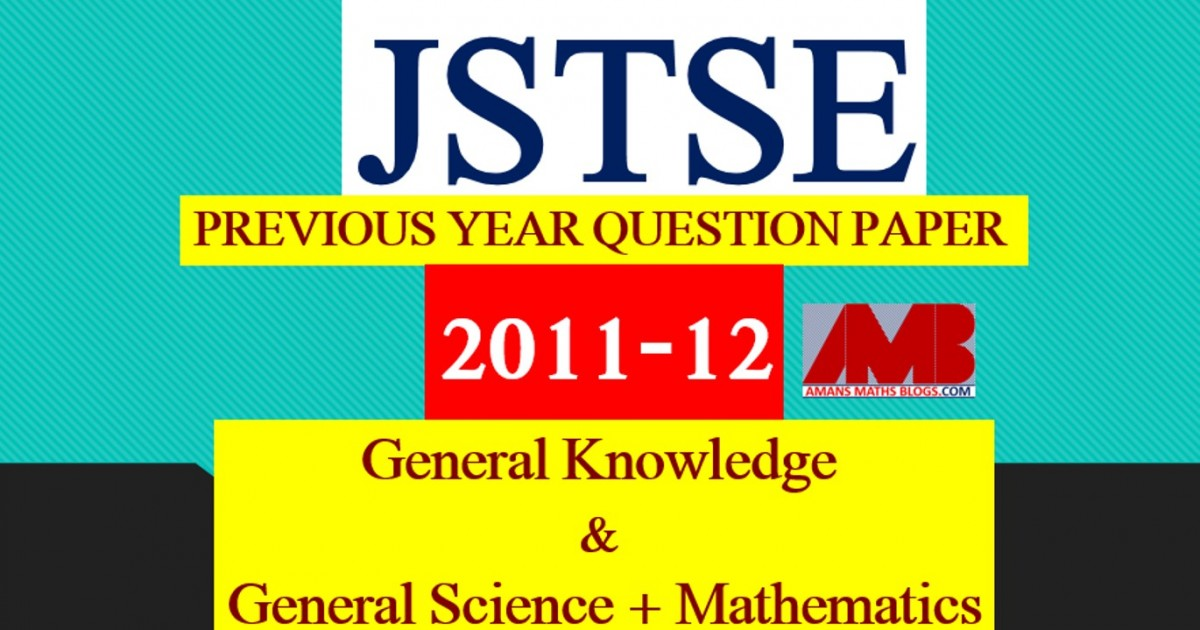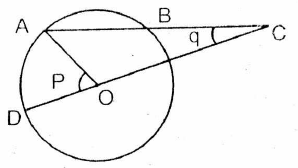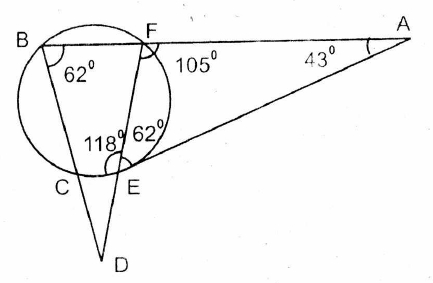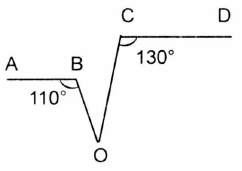Saturday, June 12, 2021
Home > download jstse papers > JSTSE PREVIOUS YEAR QUESTION PAPER 2011-12

# JSTSE PREVIOUS YEAR QUESTION PAPER 2011-12# JSTSE PREVIOUS YEAR QUESTION PAPER 2011-12

Junior Science Talent Search Examination (JSTSE) is conducted by Science Branch of DTE of Education, Delhi every year usually in the month of January for the students of recognized schools of Delhi. In this post, you will get JSTSE Previous Year Question Paper 2011-12.

JSTSE
Previous Year Question Papers
2007-082008-092009-102010-112011-12
2012-132013-142014-152015-162016-17
2018-19PhysicsChemistryMathsBiology

Eligibility: – Any student of recognised school studying in Class IX in Govt./Govt. Aided/Public/KV/Navodaya/NDMC and must have secured 65% marks in class VIII.
Examination fee: – No examination fee is charged from students for appearing in this exam.
Type of Examination: – The examination consists of two papers.
I. General Knowledge
No. of Questions: 50       Marks : 50     Time Alloted: 50 min
50 50 50 min.
II . General Science and Mathematics
No. of Questions : 150      Marks : 150     Time Alloted: 150 min

No. of Scholarships:- There are 150 scholarships for JSTSE per year.

## General Science + Mathematics

Click Here To Download This File

JSTSE Previous Year Question Paper 2011-12 Ques No 1:

Simplify : 6 1/7 + 5 1/4 + 8 1/2 – 6 1/4 = ?

Options:

A. 13 1/7

B. 6 1/5

C. 23 1/7

D. 29

JSTSE Previous Year Question Papers 2011-12 Ques No 2:

30% of 150 + ? % of 300 = 40% of 450

Options:

A. 65

B. 35

C. 45

D. 125

JSTSE Previous Year Question Paper 2011-12 Ques No 3:

In triangle ABC, AB = 3 cm, BC = 5 cm, AC = 4 cm, then the right angle will be formed at

Options:

A. Vertex A

B. Vertex B

C. Vertex C

D. None of Above

JSTSE Previous Year Question Papers 2011-12 Ques No 4:

Find the wrong term in the following series : 9359, 1559, 311, 17, 25, 12, 12

Options:

A. 77

B. 25

C. 12

D. 1558

JSTSE Previous Year Question Paper 2011-12 Ques No 5:

The difference between 31% and 12% of a number is 576. Find 17% of the same number.

Options:

A. 640

B. 888

C. 544

D. 442

JSTSE Previous Year Question Papers 2011-12 Ques No 6:

If the selling price of 10 pens is same as cost price of 8 pens, then loss % is

Options:

A. 15%

B. 20%

C. 8%

D. 30%

JSTSE Previous Year Question Paper 2011-12 Ques No 7:

In a mixture of 75 litres, the ratio of milk of water is 2 : 1. The amount of water to be further added to the mixture so as to wake the ratio of milk to water 1 : 2  will be

Options:

A. 60 lts.

B. 45 lts.

C. 75 lts.

D. 30 lts.

JSTSE Previous Year Question Papers 2011-12 Ques No 8:

The sum of the three consecutive odd numbers is 285. The smallest number is

Options:

A. 95

B. 97

C. 93

D. 99

JSTSE Previous Year Question Paper 2011-12 Ques No 9:

A sum of money doubles in 7 yrs of simple interest it will become four fold in

Options:

A. 10 yrs

B. 35 yrs

C. 21 yrs

D. 14 yrs

JSTSE Previous Year Question Papers 2011-12 Ques No 10:

By travelling at 4/5th of his usual speed a person is late by 20 minutes. Initial time to cover the distance was

Options:

A. 80 minutes

B. 65 minutes

C. 45 minutes

D. 50 minutes

JSTSE Previous Year Question Paper 2011-12 Ques No 11:

A tap can fill an empty tank in 12 hours and a leakage can empty the whole tank in  20 hours. If the tap and the leakage are working simultaneously the whole tank to fill will take

Options:

A. 25 hrs

B. 40 hrs

C. 30 hrs

D. 35 hrs

JSTSE Previous Year Question Papers 2011-12 Ques No 12:

Ratio of angle of a quadrilateral is 1 : 2 : 3 : 4. The sum of twice the smallest angle and half of the largest angle is

Options:

A. 144 degree

B. 150 degree

C. 156 degree

D. 162 degree

JSTSE Previous Year Question Paper 2011-12 Ques No 13:

Difference of circumference of two circle is 132 cm. Radius of smaller circle is 14 cm. The radius of the larger circle is

Options:

A. 35 cm

B. 40 cm

C. 46 cm

D. 54 cm

JSTSE Previous Year Question Paper 2011-12 Ques No 14:

Raj, Ritik and Piyush begin to jog around a circular stadium. They complete their revolutions in 42 sec, 56 sec and 63 sec respectively. They will be together at starting points after:

Options:

A. 336 sec

B. 504 sec

C. 252 sec

D. Can’t be determined

JSTSE Previous Year Question Paper 2011-12 Ques No 15:

Rasmi sets alarm in two different clocks. The first alarm rings after every 30 minutes and the second rings after every 90 minutes. If they ring together at 11 pm. They will ring again together at:

Options:

A. 12 : 30 am

B. 2 : 00 am

C. 1 : 30 am

D. 1 : 00 am

JSTSE Previous Year Question Paper 2011-12 Ques No 16:

Simplifying (2n + 2n-1) / (2n-1 – 2n), we get

Options:

A. 1/2

B. -3

C. 1/2 x (2n – 1)

D. 3/2 x (2n + 1)

JSTSE Previous Year Question Paper 2011-12 Ques No 17:

The value of expression √(2√(2√(2√(2….) is

Options:

A. 2

B. 3

C. 4

D. 10

JSTSE Previous Year Question Papers 2011-12 Ques No 18:

The solution of 33k+5 x 33k+3 = 9 is

Options:

A. k = 1

B. k = -1

C. k = -5/3

D. k = 1/2

JSTSE Previous Year Question Paper 2011-12 Ques No 19:

In the figure, O is the center of the circle is BC = OD, ∠AOD = P and ∠ACO = q, thenOptions:

A. p = 3q/2

B. p = 2q

C. p = 3q

D. There is no relationship between them

JSTSE Previous Year Question Papers 2011-12 Ques No 20:

In the figure, if ∠FBD = 62 degree and ∠BAC = 43 degree, then ∠EDC will beOptions:

A. 10 degree

B. 43 degree

C. 16 degree

D. 31 degree

JSTSE Previous Year Question Paper 2011-12 Ques No 21:

If cube of surface S has a volume V, then volume of cube of surface area 2S is

Options:

A. √2 V

B. 2V

C. 2√2 V

D. 4V

JSTSE Previous Year Question Paper 2011-12 Ques No 22:

If x + 1/x = 1/4 then the value of x3 + 1/x3 would be

Options:

A. 64

B. 52

C. 32

D. 12

JSTSE Previous Year Question Papers 2011-12 Ques No 23:

(1 + tan2θ) / (1 + cot2θ) is

Options:

A. sec2θ

B. -1

C. cot2θ

D. tan2θ

JSTSE Previous Year Question Papers 2011-12 Ques No 24:

The ratio of length of a pole and its shadow is 1 : √3. The angle of elevation of the sun is

Options:

A. 90 degree

B. 60 degree

C. 45 degree

D. 30 degree

JSTSE Previous Year Question Papers 2011-12 Ques No 25:

In a triangle ABC, internal bisector of angles B and C meet at P and External bisectors of B and C meet at Q. Then, angles (BPC + BQC) isOptions:

A. 90 degree

B. 90 degree – A/2

C. 90 degree + A/2

D. 180 degree

JSTSE Previous Year Question Papers 2011-12 Ques No 26:

The radius and slant height of a cone are in the ratio 4 : 7 If the curved surface area of the cone is 792 cm2. Then radius of the cone is

Options:

A. 3 cm

B. 11 cm

C. 12 cm

D. 28 cm

JSTSE Previous Year Question Papers 2011-12 Ques No 27:

The volume of the sphere curved out of a cube of side 7cm is

Options:

A. 343π/6 cm3

B. 343π/7 cm3

C. 343π/8 cm3

D. None of these

JSTSE Previous Year Question Papers 2011-12 Ques No 28:

If asinθ = bcosθ, then the value of sec2θ is

Options:

A. a2/b2

B. b2/a2

C. b2/(a2 + b2)

D. (a2 + b2)/a2

JSTSE Previous Year Question Papers 2011-12 Ques No 29:

In the figure below, the value of ∠BOC, if AB || CD, isOptions:

A. 50 degree

B. 60 degree

C. 70 degree

D. 120 degree

JSTSE
Previous Year Question Papers
2007-082008-092009-102010-112011-12
2012-132013-142014-152015-162016-17
2018-19PhysicsChemistryMathsBiology
error: Content is protected !!